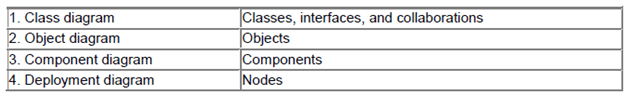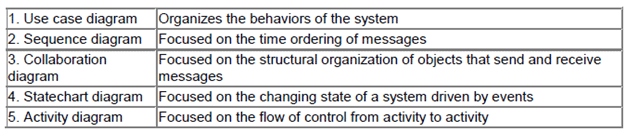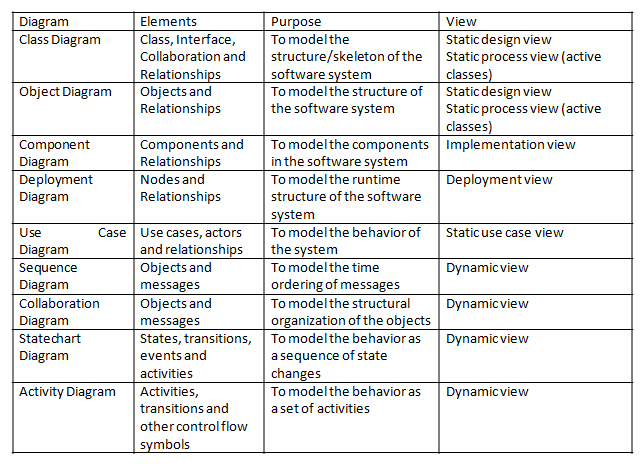UML Tutorial for Beginners
Blog containing resources for uml like lecture notes, lecture videos, lab manual, uml diagrams, objective bits, important questions and more.

09/08/2013 Categories: Structural Modeling.

In object oriented modeling we will view the software system as a collection of interacting objects. Related objects are grouped together into classes. So, every software system or application consists of classes and these classes collaborate with each other in some manner to achieve the functionality.

Modeling is all about creating a simplification or abstraction of the end software system that is going to be developed. Modeling consists of creating different diagrams.

Every diagram can be thought of as a graph containing vertices joined with edges. In UML, these vertices are replaced with things and the edges are replaced with relationships.

To better understand the system, there are different diagrams in UML. Each diagram provides different information about the system.

Every software system contains structural (static) aspects as well as behavioral (dynamic) aspects. To model the static aspects of a software system, UML provides the following diagrams:

• Class diagram
• Object diagram
• Component diagram
• Deployment diagram

To model the dynamic aspects of a software system, UML provides the following diagrams:

• Use case diagram
• Sequence diagram
• Collaboration diagram
• Statechart diagram
• Activity diagram

## Structural Diagrams

UML’s four structural diagrams allow us to model the static aspects of the software system. Static aspects of the system represent the skeleton of the system. The static aspects consist of classes, interfaces, collaborations, components and nodes.

UML’s four structural diagrams are organized around the major groups of things we will find when modeling a system:## Behavioral Diagrams

UML’s five behavioral diagrams allow us to model the dynamic aspects of the software system. The dynamic aspects can be thought of as the changing parts in the system.

The dynamics of a system can be seen as messages between objects or components moving from one place to another. UML’s behavioral diagrams are organized around the major ways you can model the dynamics of a system:All the diagrams in UML can be summarized as shown below:## Common Modeling Techniques

### Modeling different views of a system

To model a system from different views:

1)      Choose the suitable views for modeling the software system.

2)      Create the UML diagrams for each view you have chosen.

3)      Decide which diagrams will be reviewed and used in the documentation of the project.

4)      Allow room for the diagrams that are discarded.

For example, for a simple standalone centralized application may be use case view, design views are enough. But, for a complex distributed application we need all the views.

### Modeling complex views

To model complex views,

1)      First, hide the unnecessary details using the notations of UML.

2)      Still if the diagrams are complex, consider grouping the related elements using packages.

3)      Still, if the diagrams are complex, consider using notes and different colors for the elements to draw the attention of the readers.